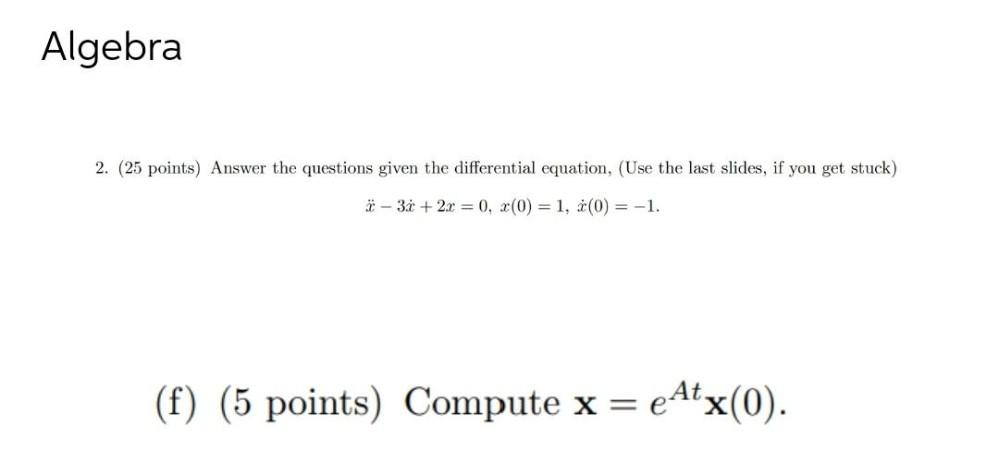Question:

# Answer the questions given the differential equation, x– 3xAnswer the questions given the differential equation, x– 3x + 2x = 0, x(0) =1, x(0) = -1. Compute x =e^Atx(0)